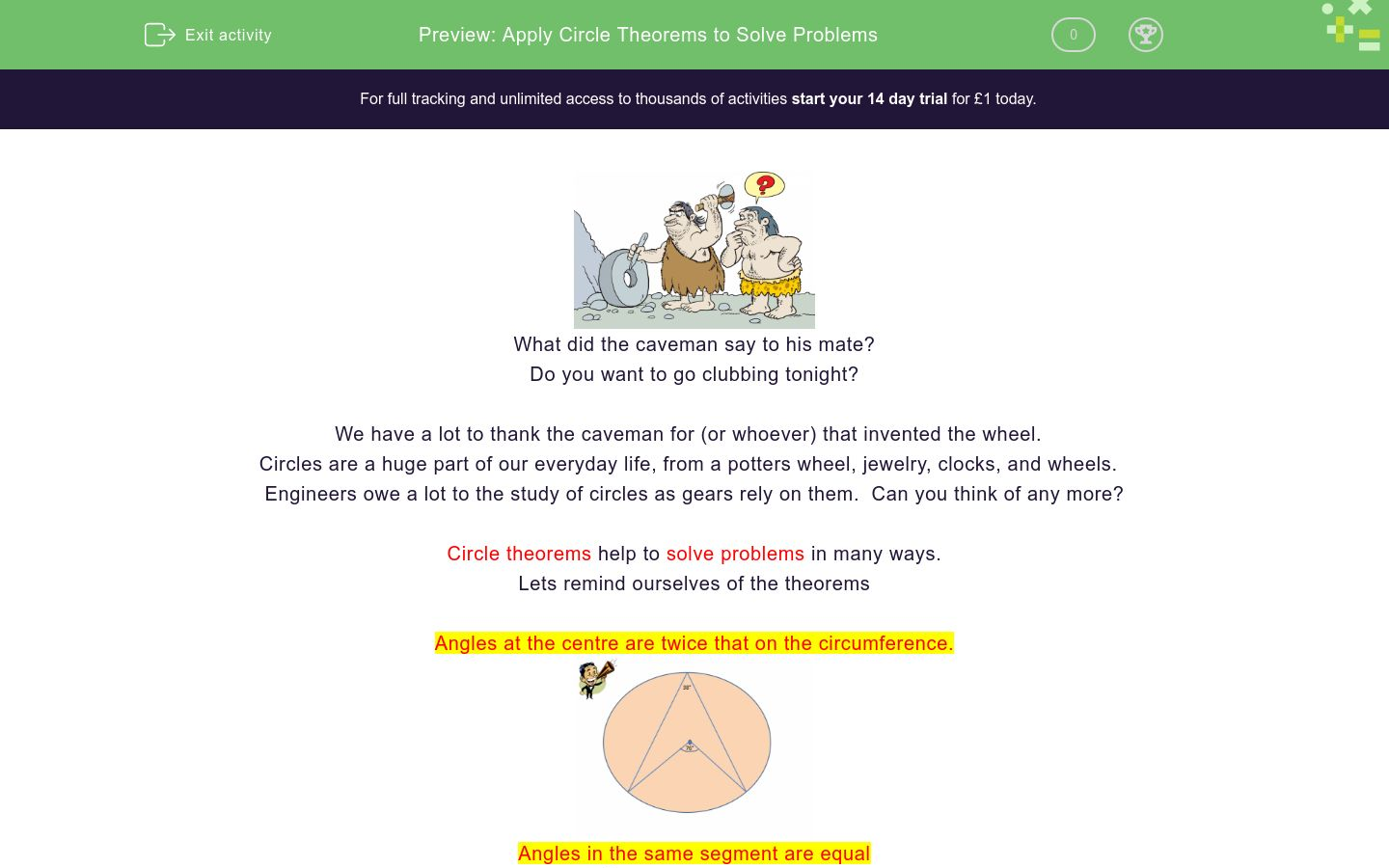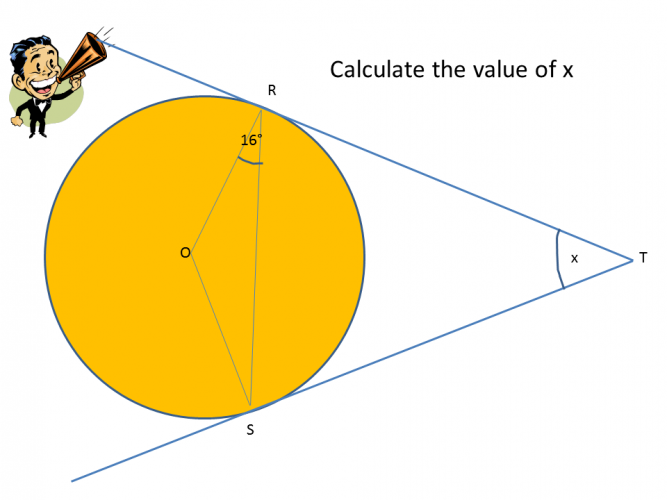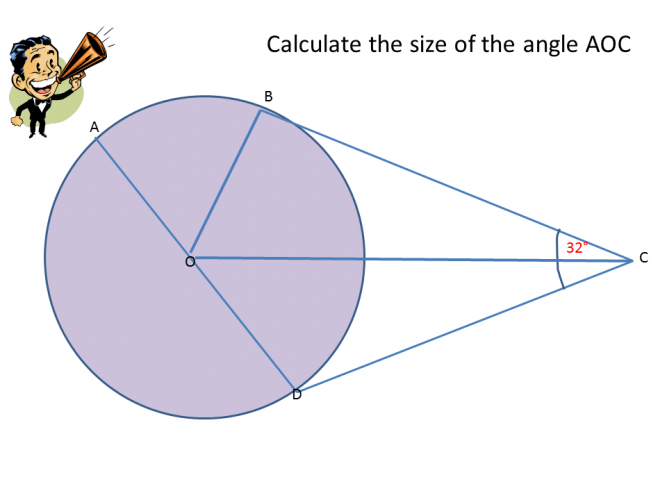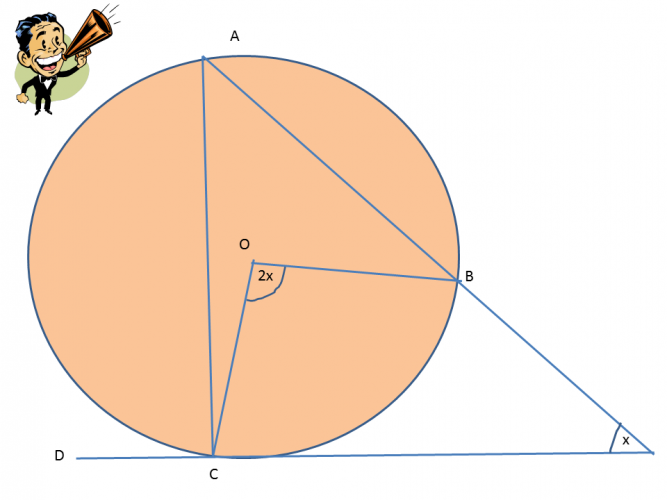# Apply Circle Theorems to Solve Problems

In this worksheet, students will apply circle theorems to solve problems.Key stage:  KS 4

GCSE Subjects:   Maths

GCSE Boards:   OCR, AQA, Eduqas, Pearson Edexcel

Curriculum topic:   Basic Geometry, Geometry and Measures

Curriculum subtopic:   Circles, Properties and Constructions

Difficulty level:### QUESTION 1 of 10What did the caveman say to his mate?

Do you want to go clubbing tonight?

We have a lot to thank the caveman for (or whoever) that invented the wheel.

Circles are a huge part of our everyday life, from a potters wheel, jewelry, clocks, and wheels.

Engineers owe a lot to the study of circles as gears rely on them.  Can you think of any more?

Circle theorems help to solve problems in many ways.

Lets remind ourselves of the theorems

Angles at the centre are twice that on the circumference.Angles in the same segment are equalAngle subtended from the diameter is 90°Tangents to a circle meet the circumference at 90° and the lines are of equal length.Angles in alternate segments are equalThe trick to being successful in these questions is to be able to spot the theorems.In problems solving questions, there is usually more than one theorem to follow.

Quite often you will have to find angles that are not identified first.

Lets investigate the magic.45°

55°

90°

65°32° 74° 48° Angle x is32° 74° 48° Angle x is90° 40° 32° 68° Angle x Angle y Angle zAngle x is 116°, 32°, 58°, 45°, 64°. Angle y is 116°, 32°, 58°, 45°, 64° and angle z is 116°, 32°, 58°, 45°, 64°Angle x is 116°, 32°, 58°, 45°, 64°. Angle y is 116°, 32°, 58°, 45°, 64° and angle z is 116°, 32°, 58°, 45°, 64°20° 35° 45° 55° 60° 70° Angle x is Angle y is Angle z23°

67°

44°

58°

• Question 165°
EDDIE SAYS
To solve this is the tangents at the circumference theorem is used. However, in this one the angle is not 90°. This is an easy trap to fall into. If you draw a line from R to S you can see that these are not right angles. This become an isosceles triangle and a straightforward angles in a triangle add up to 180° 180 - 50 = 130° 130 ÷ 2 = 65°
• Question 232° 74° 48° Angle x is
EDDIE SAYS
This is where the tangents meet the circumference at 90° can really help us. Hooray. 90 - 16 = 74° which give us angle TRS. The triangle is isosceles therefore angle TSR is also 74° 180 - 74 - 74 = 32° which is angle x.
• Question 3EDDIE SAYS
Did you spot the alternate segment theorem for angle x. Angles in alternate segments are the same. What can you remember about opposite angles in cyclic quadrilaterals? Yes they add up to 180° 180 - 76 = 104° Therefore both x and y are 104°
• Question 490° 40° 32° 68° Angle x Angle y Angle z
EDDIE SAYS
Yes I know there is quite a lot of work, but you can do it. You can work these out in any order. Angle ACB - 90° (Angles from the diameter) Angle Z can be found from angles in a triangle add up to 180°. 180 - 90 - 50 =40° x can be found in the same way now that we know z. 180 - 90 - 40 - 18 = 32° Angle ADB is 90° (angles from the diameter) Angle y can now be found 180- 90 - 32 - 18 = 40°
• Question 5Angle x is 116°, 32°, 58°, 45°, 64°. Angle y is 116°, 32°, 58°, 45°, 64° and angle z is 116°, 32°, 58°, 45°, 64°
EDDIE SAYS
All you need here is your eagle eye and to draw on your basic knowledge of angles and triangles. Angle ABC is 90° (angle from the diameter) AOB forms an isosceles triangle therefore angle ABO also 32° x = 58° (90 - 32) Angle AOB 180 - 32 - 32 = 116°: y = 64° (Angles on a straight line 180 - 116) Angle ADC (angle from the diameter 90°) The triangle formed is isoceles. Therefore 180 - 90 = 90° 90° ÷ 2 = 45° z = 45°
• Question 6EDDIE SAYS
This is not so tricky as it looks. Did you spot that x is the angle on the circumference from 82°, therefore it is half 41° Angle y is in the same segment as x so also 41° To find angle z find angle AOD (180 - 82 = 98°) 180 - 98 - 41= 41° also. I love these investigations don't you.
• Question 758
EDDIE SAYS
Always look at what you know first and mark it on the diagram. You may use some of the information you have put in you may not use it all. Line OB is perpendicular to line BC so angle OBC is 90° Therefore angle AOC = 180 - 90 - 32 = 58°
• Question 8EDDIE SAYS
Are you getting used to spotting these now? Of course, so no numbers, no problem. You can only work with what you have so Angle BAC is x as angle at the centre is twice that on the circumference. Angle DCA is 2x (alternate angle segment) Angle ACO is 90 - 2x (Angles at a tangent)
• Question 920° 35° 45° 55° 60° 70° Angle x is Angle y is Angle z
EDDIE SAYS
Angle x is 70° as the angle at the centre is twice that on the circumference. Angle y is 20°, (Angles in a triangle) Angle z is 55°, (BEC is 35°, angles in the same segment, BCE is 90° angles at a tangent and finally angles in a triangle add up to 180° 180 - 90 - 35 = 55°)
• Question 1044°
EDDIE SAYS
Oh, so quite a bit to do here but you have all the knowledge. Angle STO = 23° Angle OST = 90° so angles in a triangle 180 - 90 - 23 = 67°. Angle RTO = 23° Angle ORT = 90° (angles at a tangent) Angle BOT = 90 - 67 = 23° Therefore Angle ROB = 180 - 90 - 23 - 23 = 44°.
---- OR ----

Sign up for a £1 trial so you can track and measure your child's progress on this activity.

### What is EdPlace?

We're your National Curriculum aligned online education content provider helping each child succeed in English, maths and science from year 1 to GCSE. With an EdPlace account you’ll be able to track and measure progress, helping each child achieve their best. We build confidence and attainment by personalising each child’s learning at a level that suits them.

Get started